# Matching Pi Attenuator Calculator

Calculates the resistor values, attenuation, minimum attenuation, 'impedance', reflection coefficient, VSWR and return loss of a matching Pi attenuator. This can be built into a FLEXI-BOX and a transmission line (50 Ohm track) PCB is available which easily adapts for this circuit with one simple trimming operation. There is also a selection of 50 Ohm RF connectors available.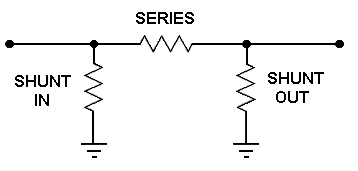1) Enter the required input impedance, output impedance and attenuation then press "Calculate" below to obtain the Ideal resistor values.

 INPUT DATA Input Impedance: Ohms Output Impedance: Ohms Attenuation: dB
 IDEAL VALUES Ideal Shunt In resistor: Ohms Ideal Shunt Out resistor: Ohms Ideal Series resistor: Ohms Minimum attenuation to achieve impedance match: dB

2) Change the calculated Ideal resistor values in the amber text boxes below to the nearest Preferred Resistor Values or to those available then press " Calculate" below to get the resulting attenuator parameters.

 SELECTED VALUES Shunt In resistor: Ohms Shunt Out resistor: Ohms Series resistor: Ohms Mismatch Losses: Included (see note below)
 PARAMETERS Forward Attenuation: dB Input Impedance: Ohms Input VSWR: Input Reflection Coefficient: Input Return Loss: dB
 Reverse Attenuation: dB Output Impedance: Ohms Output VSWR: Output Reflection Coefficient: Output Return Loss: dB

This calculator uses JavaScript and will function in most modern browsers. For more information see About our calculators

The required input and output impedance are used to calculate the minimum attenuation possible for correct impedance matching with an equation from RF Design Guide, Systems, Circuits and Equations by Peter Vizmuller Published by Artech House ISBN 0-89006-754-6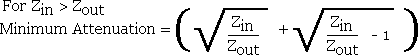Where Zin is the input system characteristic impedance

Zout is the output system characteristic impedance

Peter Vizmuller also gives equations for calculating the resistor values directly. This calculator uses equations that I have derived and first finds the output voltage with a source EMF of 2 Volts and therefore an input PD of 1 Volt.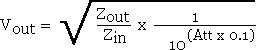The individual ideal resistor values can now be found.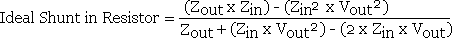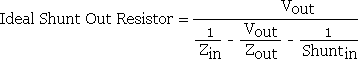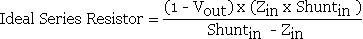After selecting preferred values, simple series and parallel resistor calculations are used to find the attenuator input impedance, input voltage and output voltage. The source EMF is again assumed to be 2 Volts.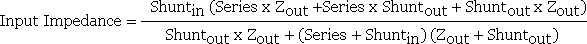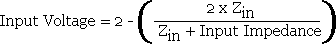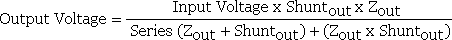The remaining equations for Attenuation, Voltage Reflection Coefficient and VSWR are available at:-

http://www.spectrum-soft.com/news/fall2009/vswr.shtm

Note: With the Mismatch Losses box ticked the calculator will return an attenuation calculated from the source power in relation to the attenuator output power which is suitable for calculations at RF and Microwave frequencies, particularly if the attenuator is located away from the source at the end of a transmission line. Without the Mismatch Losses box ticked the calculator will return an attenuation calculated from the actual input power to the attenuator in relation to the output power which is more suitable for calculations at audio frequencies. The difference in attenuation with the two methods is only noticeable when there is a significant mismatch.

This calculator is provided free by Chemandy Electronics in order to promote the FLEXI-BOX